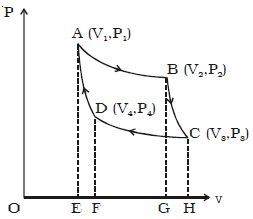Physics

# Isothermal Compression of Carnot Cycle

Isothermal compression of Carnot Cycle

The cylinder is placed on the sink at a temperature T2. The piston is moved slowly downward to compress the gas isothermally. This is represented by CD. Let (V4, P4) be the volume and pressure corresponding to the point D. Since the base of the cylinder is conducting the heat produced during compression will pass to the sink so that, the temperature of the gas remains constant at T2. Let Q2 be the amount of heat rejected to the sink and W3 be the amount of work done on the gas in compressing it isothermally.Q2 = W3 = v4v3 – PdV = – RT2 loge (V4/V3) = – Area CDFHC

The negative sign indicates that work is done on the working substances.

So, Q2 = RT2 loge (V3/V4)